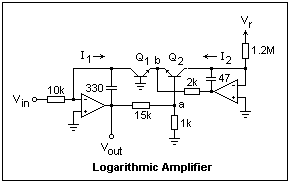## Logarithmic Amplifier

In a linear amplifier, the input V and output V' voltages are related by V' = GV + B, where G is the gain. In a logarithmic amplifier, they are related by V' = A log V + B. It is not as hard to make a logarithmic amplifier as it might seem, because we have a very accurate exponential relationship between the collector current I and base-to-emitter voltage VBE: I = Io exp(qVbe/kT), or Vbe = (kT/q) ln (I/Io). Logarithms to different bases are proportional, so we can easily change bases if it is convenient. In particular, log x = 0.4343 ln x, or ln x = 2.3026 log x.

The difference in the base-emitter voltages of two transistors is Vbe1 - Vbe2 = (kT/q) [ln (I1/I2) + ln (Io2/Io1)]. If we can hold I2 constant, then the difference is proportional to ln I. We can easily make a current proportional to a voltage, so our problem is solved.A circuit that does just that is shown in the diagram at the right, simplified down to the essentials. We use two op-amps, each with a virtual ground at the inverting input, where we create the currents I1 and I2, one from the input voltage and one from a reference voltage, here +12. The reference current is 10 μA. A 412 is a good choice for the op-amp, since it is a dual, and the bias currents are very small, so they do not have to be considered. The op-amps maintain the collector currents at the desired values. The 330 pF and 47 pF capacitors only have the function of ensuring stability at high frequencies. The two transistors are 2N4124's--I just happened to have them handy. If you were making a serious logarithmic amplifier, you might want to use a specially-matched pair of transistors like the LM394. However, the 2N4124's will work just fine, and we can see what a little mismatch would cause.

The key to the circuit is node "a" where the voltage is Vbe2 - Vbe1. The output voltage is simply a multiple of this voltage, here 16 times, but in general (R1 + R2/R2. The ratio of the collector currents is (VinR3)/(VrR4), or 10Vin, with the values shown. If we substitute all these numbers in the formula, using 0.025 V for the thermal voltage, we find Vout = -0.4 ln Vin - 0.921 + 0.4 ln (Io1/Io2). Note how the factor 10 and the difference in Io's affect the expression. By properly choosing values, and using matched transistors, we can get rid of the constant term. It's not worth the bother here, and we learn a little more anyway.

Now build the circuit and test it, using a buffered potentiometer for the input voltage, as usual. Plot the output voltage as a function of the natural logarithm of the input voltage. I got a very nice straight line from only seven points, and the formula Vout = -0.44 ln Vin - 0.90 (hardly accurate to two places), which agrees nicely with the expected equation. The thermal voltage is a little higher than 0.025 V anyway, since this is August. The input current varied from 1 mA down to 0.5 μA in the test.

With a logarithmic amplifier, you can create a meter that reads directly in dB, or handle signals with a large dynamic range. It is interesting that this circuit works so well, in any case. The circuit can be dressed up with zero adjustments and other niceties.